Author: Oscar Cronquist Article last updated on May 05, 2022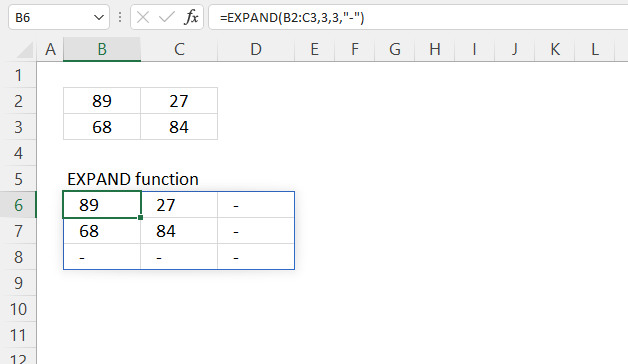The EXPAND function increases a cell range or array by a specified number of columns and rows.

Dynamic array formula in cell B6:

=EXPAND(B2:C3, 3, 3, "-")

The EXPAND function is available to Excel 365 users and is in the "Array manipulation" category.

## 2. EXPAND Function Arguments

 array Required. The source cell range or array. rows Required. The new number of rows. [columns] Optional. The new number of columns. [pad_with] Optional. A string to pad new array values with. Default is #N/A meaning not available.

## 3. EXPAND Function example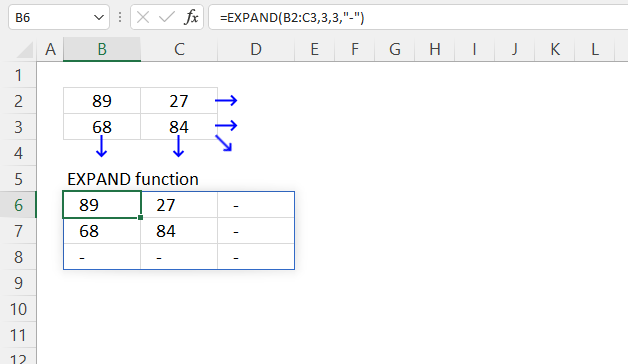The picture above shows how the EXPAND function increases the original array from two columns and two rows to three columns and three rows.

The number of cells increases to 9 from 4 cells, 3 rows * 3 columns equals 9 cells. The new cells are padded with a minus sign.

Dynamic array formula in cell B6:

=EXPAND(B2:C3, 3, 3, "-")
Note! If any of the values in the source range change, the values in the EXPAND function output change as well.

### 3.1 Explaining formula

#### Step 2 - Populate arguments

array - B2:C3
rows - 3
[columns] - 3
[pad_with] - "-". The double quotes are needed if a non-numeric value is used.

#### Step 3 - Evaluate function

EXPAND(B2:C3, 3, 3, "-")

becomes

EXPAND({89, 27;
68, 84}, 3, 3, "-")

and returns

{89, 27, "-";
68, 84, "-";
"-", "-", "-"}

## 4. EXPAND Function example - how to extend an array up and left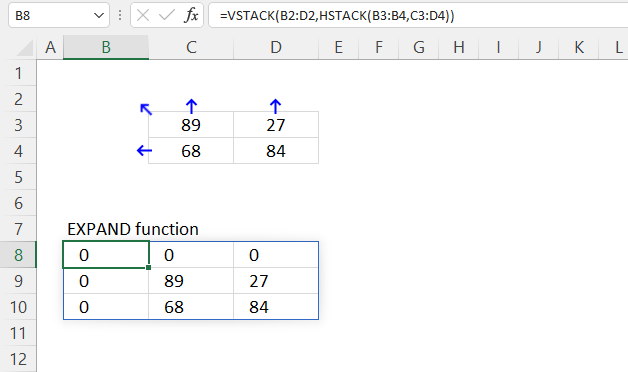The EXPAND function lets you add more rows and columns to an array, they are, however, added to the right and below of the source array.

Use the following formula to add array containers above and to the left of the original array.

Dynamic array formula in cell B8:

=VSTACK(B2:D2, HSTACK(B3:B4, C3:D4))

### Explaining formula

#### Step 1 - Add arrays horizontally

The HSTACK function lets you combine cell ranges or arrays, it joins data to the first blank cell to the right of a cell range or array (horizontal stacking)

HSTACK(array1, [array2],...)

HSTACK(B3:B4, C3:D4)

becomes

HSTACK({0; 0}, {89, 27; 68, 84})

and returns

{0, 89, 27; 0, 68, 84}.

#### Step 2 - Add arrays vertically

The VSTACK function lets you combine cell ranges or arrays, it joins data to the first blank cell at the bottom of a cell range or array (vertical stacking).

VSTACK(array1, [array2],...)

VSTACK(B2:D2, HSTACK(B3:B4, C3:D4))

becomes

VSTACK({0, 0, 0}, {0, 89, 27; 0, 68, 84})

and returns

{0, 0, 0;
0, 89, 27;
0, 68, 84}.

## 5. EXPAND Function example - extend array dynamically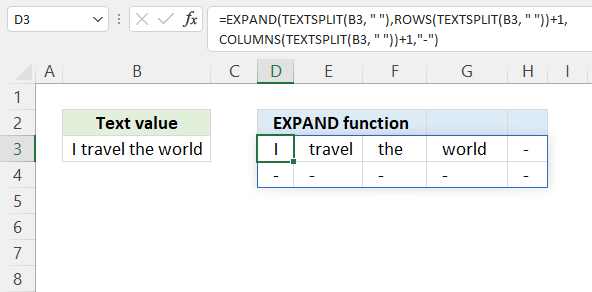The section shows how to return dynamically resize an array meaning the array is always one row and one column larger than the source array.

Try changing the text value in cell B3 and the dynamic array formula in cell D3 adjusts accordingly.

Dynamic array formula in cell B9:

=LET(x, TEXTSPLIT(B3, " "), EXPAND(x, ROWS(x)+1, COLUMNS(x)+1, "-"))

### Explaining formula

EXPAND(TEXTSPLIT(B3, " "), ROWS(TEXTSPLIT(B3, " "))+1, COLUMNS(TEXTSPLIT(B3, " "))+1, "-")

#### Step 1 - Split text

The TEXTSPLIT function lets you split a string into an array across columns and rows based on delimiting characters.

TEXTSPLIT(Input_Textcol_delimiter, [row_delimiter], [Ignore_Empty])

TEXTSPLIT(B3, " ")

#### Step 2 - Calculate rows in array and add 1

The ROWS function calculates the number of rows in a given cell range or array.

ROWS(array)

ROWS(TEXTSPLIT(B3, " "))+1

#### Step 3 - Calculate columns in array and add 1

The COLUMNS function calculates the number of columns in a given cell range or array.

COLUMNS(array)

COLUMNS(TEXTSPLIT(B3, " "))+1

#### Step 4 - Expand array one row and one column

EXPAND(TEXTSPLIT(B3, " "), ROWS(TEXTSPLIT(B3, " "))+1, COLUMNS(TEXTSPLIT(B3, " "))+1, "-")

becomes

EXPAND({"I", "travel", "the", "world"}, 1+1, 4+1, "-")

and returns

{"I", "travel", "the", "world", "-";
"-", "-", "-", "-", "-"}

#### Step 5 - Shorten the formula

The LET function lets you name intermediate calculation results which can shorten formulas considerably and improve performance.

LET(name1name_value1calculation_or_name2, [name_value2calculation_or_name3...])

TEXTSPLIT(B3, " ") is repeated three times.

EXPAND(TEXTSPLIT(B3, " "), ROWS(TEXTSPLIT(B3, " "))+1, COLUMNS(TEXTSPLIT(B3, " "))+1, "-")

becomes

LET(x, TEXTSPLIT(B3, " "),EXPAND(x,ROWS(x)+1,COLUMNS(x)+1,"-"))

## 6. EXPAND function error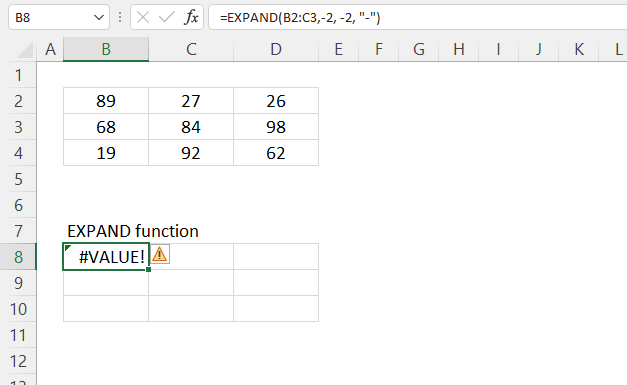The EXPAND function returns a #VALUE! error if a negative number is used in the second or third argument.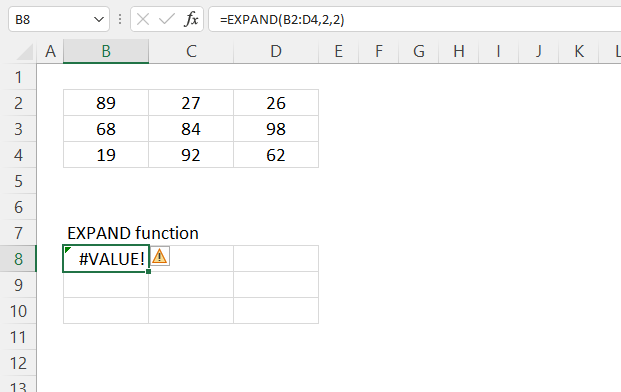Numbers smaller than the actual array size also return  a #VALUE! error. Cell range B2:D4 has three rows and three columns.

## 7. EXPAND function - values in random order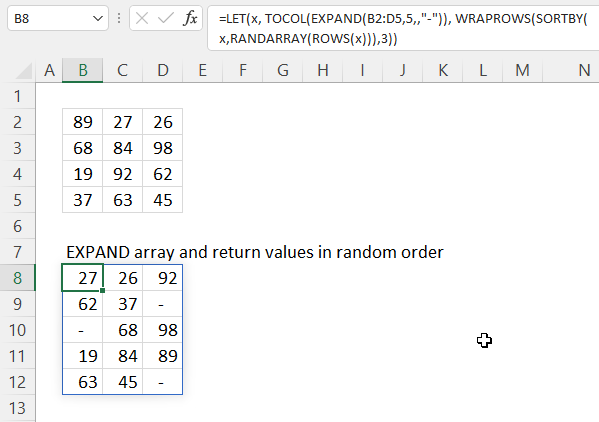The image above demonstrates a formula that adds an additional row to cell range B2:D5 and the returns values in random order.

Dynamic array formula in cell B8:

=LET(x, TOCOL(EXPAND(B2:D5, 5, , "-")), WRAPROWS(SORTBY(x, RANDARRAY(ROWS(x))), 3))

### 8.1 Explaining formula in cell B8

WRAPROWS(SORTBY(TOCOL(EXPAND(B2:D5,5,,"-")),RANDARRAY(ROWS(TOCOL(EXPAND(B2:D5,5,,"-"))))),3)

#### Step 1 - Expand array with an extra row

EXPAND(B2:D5,5,,"-")

becomes

EXPAND({89, 27, 26;68, 84, 98;19, 92, 62;37, 63, 45}, 5, , "-")

and returns

{89, 27, 26;
68, 84, 98;
19, 92, 62;
37, 63, 45;
"-", "-", "-"}

#### Step 2 - Rearrange values into a single column

The TOCOL function rearranges values from a 2D cell range or array to a single column.

TOCOL(array, [ignore], [scan_by_col])

TOCOL(EXPAND(B2:D5,5,,"-"))

becomes

TOCOL({89, 27, 26;
68, 84, 98;
19, 92, 62;
37, 63, 45;
"-", "-", "-"})

and returns

{89; 27; 26; 68; 84; 98; 19; 92; 62; 37; 63; 45; "-"; "-"; "-"}

The semicolon is a row delimiting character, your computer may use an other row delimiting character.

#### Step 3 - Count cells

The ROWS function returns the number of columns in a given cell range or array.

ROWS(array)

ROWS(TOCOL(EXPAND(B2:D5,5,,"-")))

becomes

ROWS({89; 27; 26; 68; 84; 98; 19; 92; 62; 37; 63; 45; "-"; "-"; "-"})

and returns 15.

#### Step 4 - Create random cells

The RANDARRAY function returns a table of random numbers across rows and columns.

RANDARRAY([rows], [columns], [min], [max], [whole_number])

RANDARRAY(ROWS(TOCOL(EXPAND(B2:D5, 5, , "-")))))

becomes

RANDARRAY(15)

and returns

{0.215398134613085, 0.390607168196479, ... ,0.83231474462401}.

#### Step 6 - Rearrange values in random order

The SORTBY function allows you to sort values from a cell range or array based on a corresponding cell range or array.

SORTBY(array, by_array1, [sort_order1], [by_array2, sort_order2],…)

SORTBY(TOCOL(EXPAND(B2:D5, 5, , "-")), RANDARRAY(ROWS(TOCOL(EXPAND(B2:D5, 5, , "-")))))

becomes

SORTBY({89; 27; 26; 68; 84; 98; 19; 92; 62; 37; 63; 45; "-"; "-"; "-"}, {0.215398134613085, 0.390607168196479, ... ,0.83231474462401})

and returns

{"-"; "-"; 84; 62; 89; 92; 98; 37; 63; "-"; 26; 27; 45; 68; 19}.

#### Step 7 - Rearrange values to the original array size

The WRAPROWS function rearranges values from a single row to a 2D cell range based on a given number of values per row.

WRAPROWS(SORTBY(TOCOL(EXPAND(B2:D5,5,,"-")),RANDARRAY(ROWS(TOCOL(EXPAND(B2:D5,5,,"-"))))),3)

becomes

WRAPROWS({"-"; "-"; 84; 62; 89; 92; 98; 37; 63; "-"; 26; 27; 45; 68; 19},3)

and returns

{84,92,"-";
"-",68,63;
27,37,19;
45,62,"-";
98,89,26}

#### Step 8 - Shorten formula

The LET function lets you name intermediate calculation results which can shorten formulas considerably and improve performance.

LET(name1name_value1calculation_or_name2, [name_value2calculation_or_name3...])

WRAPROWS(SORTBY(TOCOL(EXPAND(B2:D5,5,,"-")),RANDARRAY(ROWS(TOCOL(EXPAND(B2:D5,5,,"-"))))),3)

becomes

LET(x, TOCOL(EXPAND(B2:D5, 5, , "-")), WRAPROWS(SORTBY(x, RANDARRAY(ROWS(x))), 3))